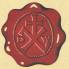Wacker Art Rotation MatricesBild: "Alter Leuchturm Travemünde"

Attention

This page has been tested and developed with the Mozilla browser. This page requires JavaScript in your browser. Without JavaScript or JavaScript beeing disabled, the display of this side is incomplete.

Prolog

Jetzt geht es rund; sprach der Papagei und flog in den Ventilator!

Rererences

This page is based on the concepts developed on my geometric algebra page and on my linear algebra page.

Reflection and Rotation

Reflection of a vector v.

Reflection and rotation of a vector v, can be expressed as a the matrix product with this vector and special matrices. The result of this product is the reflected or rotated vector. In the following graphics the original vector is drawn in black. The vector is drawn in green after the application of the G matrix, in red after the application of the R matrix and in blue after the application of the B matrix.

Reflection at the x-axis

Multipying the vector v with the G matrix will result in a reflection of the original vector at the x-axis.

Reflection at y=x Line

Multipying the vector v with the R matrix will result in a reflection of the original vector at the x=y line.

Rotation by 90° in the Counter Clockwise Direction

The combination of a reflection at the x-axis and the reflextion at the y-axis will result in a rotation by 90 degree in the counter clockwise direction (right handed rotation).

B = RG

Two Reflections and a 90° Degree Rotation

The three example operations from above in one diagram:

Rotation by 90° in the Counter Clockwise Direction - Right Handed Rotations by 90°

Applying the righthanded rotation by the B matrix several times will result in the following rotations:

 b = Bv b = B2v b = B3v b = B4v

Rotation by 90° in the Clockwise Direction - Left Handed Rotations by 90°

The left handed rotation or clockwise rotation by 90° is performend by the L Matrix L = -B

The combination of a reflection at the y-axis and the reflextion at the x-axis will result in a rotation by 90 degree in the clockwise direction (left handed rotation).

L = GR

Example:

Applying the lefthanded rotation by the L matrix several times will result in the following rotations:

 b = Lv b = L2v b = L3v b = L4v

Group Properties

The following four matrices form a group.

B2 = -I;    R2 = I;     G2 = I

B = RG;    R = BG;    G = RB;

-B = GR;   -R = GB;   -G = BR;

Perfoming 4 times a rotation by 90° will result in the Identity Matrix: B4 = I.

Details:

The Relation to the geometric algebra G2 can be found on the geometric algebra and matrices page:

Relation to Complex Numbers

Complex numbers z can be represented by the following matrix equation: z = xI + yB.

The abstract definition of a group can be found here:

Rotation Matrices

If R is a n×n matrix and the value of the determinant of R; det(R) = 1; then R is a rotation matrix.

Multiplying the rotation matrix R with the vector v which has n elements will result in a vector v'.

v' = Rv

A rotation matrix R change only the direction of a vector v when this vector is multiplied with the matrix R and leave the length of a the vector v unchanged:

|v'| = |Rv| = |v|

2×2 Rotation Matrices

Let the rotation matrix have the following form:

Special Orthogonal Group SO2

These rotation matrices R form the special orthogonal group SO2. The elements of these groups are the points of the unit circle in the plane.

Rotation Examples

Application of Different Rotation Matrices on Vectors

Unit vectors e1 and e2:

Application of the unit vectors e1 and e2 on the general rotation matrix R(θ):

Application of different rotation matrices on the vectors e1 and e2:

Rotations in Three Dimensions

Rotation Around the z-axis

The next page is about Geometric Algebra.

18. Dezember 2017 Version 1.0
Copyright: Hermann Wacker Uhlandstraße 10 D-85386 Eching bei Freising Germany Haftungsausschluß